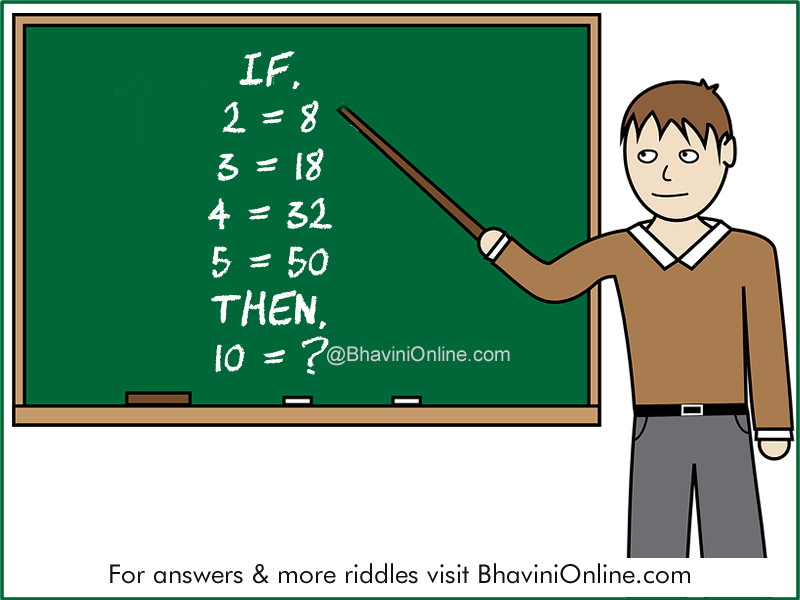# Numerical Riddle: If 2 = 8 Then, 10 = ?

Look at the given sets of equations in the riddle and find the connection between them.

Find in the missing number in the last equation;

If,
2 = 8
3 = 18
4 = 32
5 = 50
Then,
10 = ?So were you able to solve the riddle? Leave your answers in the comment section below.